Courses

# Turing Machine (TM) Computer Science Engineering (CSE) Notes | EduRev

## Computer Science Engineering (CSE) : Turing Machine (TM) Computer Science Engineering (CSE) Notes | EduRev

The document Turing Machine (TM) Computer Science Engineering (CSE) Notes | EduRev is a part of the Computer Science Engineering (CSE) Course Theory of Computation.
All you need of Computer Science Engineering (CSE) at this link: Computer Science Engineering (CSE)

Turing Machines
Consider the following figure: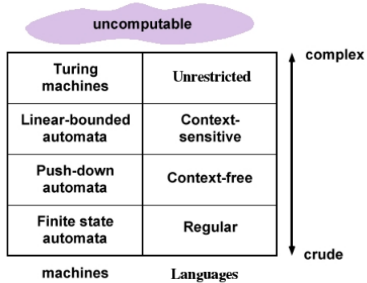From the diagram, unrestricted grammars produce unrestricted languages (Type-0 languages). These Type-0 languages are recognised using Turing machines.

Following is an example for unrestricted grammar:
S → ACaB
CaBc → aaC
CB → DB
aEC → Ea
AE → ε
Here LHS and RHS can contain any set of terminals and non terminals.

Part I. Turing Machine (TM)
A Turing machine is a computing device that can be represented as,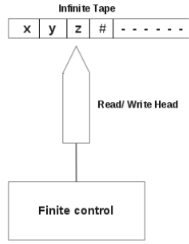From the above diagram, a TM consists of a,
1. Tape
It is divided into a number of cells. Tape is infinite. A cell can hold a tape symbol or a blank symbol (#).
2. Finite control
At a particular instant, finite control is in a state. States are divided into,
Start state (q0),
Halt state (h),
Intermediate states (q1, q2, .....).
Tape head points to one of the tape cells. It communicates between finite control and tape. It can move left ,right or remain stationary.

1 Definition of a TM
A Turing machine is,
TM = (Q,∑, Γ, δ, q0, #, h)
where
Q is a set of states,
∑ is a set of input symbols,
Γ is a set of tape symbols, including #,
δ is the next move function from
(Q × Γ) → (Q × Γ × {L, R, N}),
q0 is the start state,
# is the blank symbol,
h is the halt state.

Example:
Consider the following TM,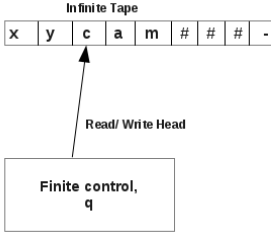Here TM is in state, q and head points to tape symbol, c.
Let a move is defined as,
δ(q, c) = (q1, b, R)
This means, if TM is in state q and points to input symbol, c, then it enters state, q1, replaces symbol, c with b and moves one position towards right (R). Thus the TM now is,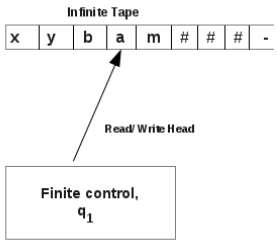Now TM is in state q1,and head points to the tape symbol, a.

Let a move of the TM is defined as,
δ(q1, a) = (q2, d, L)
This means, if TM is in state q1 and points to input symbol, a, then it enters state, q2, replaces symbol, a with d and moves one position towards left (L). Thus the TM now is,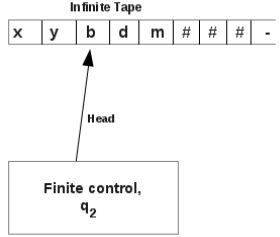Now TM is in state q2,and head points to the tape symbol, b.

Let a move of the TM is defined as,
δ(q2, b) = (q1, y, N)
This means, if TM is in state q2 and points to input symbol, b, then it enters state, q1, replaces symbol, b with y and head
remains in the same position (N). Thus the TM now is,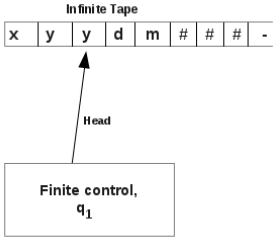Now TM is in state q1,and head points to the tape symbol, y.

Example:
Consider a Turing machine,
TM = (Q,∑, Γ, δ, q0, #, h)
where
Q = {q0, h}
∑= {a, b}
Γ = {a, b, #}
q0 = q0
# = #
h = h
δ is defined as,
δ(q0, a) = (q0, #, L)
δ(q0, b) = (q0, #, L)
δ(q0, #) = (h, #, N)
δ(h, #) = ACCEP T

Language Acceptability by TM
A string, w is said to be accepted by a Turing machine, if TM halts in an accepting state. That is,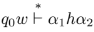A string, w is not accepted by a TM, if TM halts in a non-accepting state or does not halt.

Example 1:
Consider a Turing machine,
TM = (Q,∑, Γ, δ, q0, #, h)
where
Q = {q1, q2, q3, q4, q5, q6}
∑ = {0, 1, x, y}
Γ = {0, 1, x, y, #}
q= q1
# = #
h = q6
δ is defined as,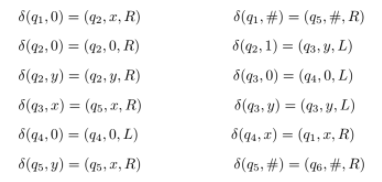Check whether the string 011 is accepted by the above TM?

Initially, TM is in start state, q1 and the tape contains the given string 011. Initially, head points to symbol 0 in the input string.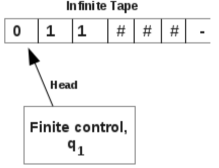A given transition is, δ(q1, 0) = (q2, x, R)
Then, TM becomes,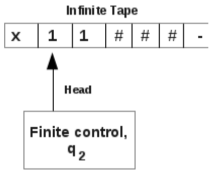A given transition is, δ(q2, 1) = (q3, y, L)
Then, TM becomes,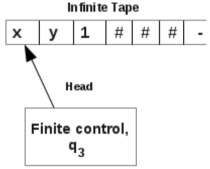A given transition is, δ(q3, x) = (q5, x, R)
Then, TM becomes,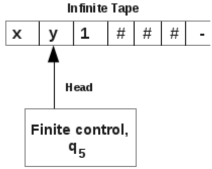A given transition is, δ(q5, y) = (q5, x, R)
Then, TM becomes,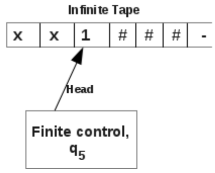δ(q5, 1) is not defined for the TM. So the string 011 is not accepted by the above TM.

Example 2:
Consider a Turing machine,
TM = (Q,∑, Γ, δ, q0, #, h)
where
Q = {q1, q2, q3, q4, q5, q6}
∑= {0, 1, x, y}
Γ = {0, 1, x, y, #}
q0 = q1
# = #
h = q6
δ is defined as,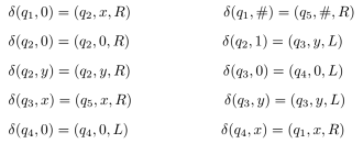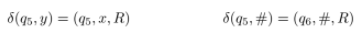Check whether the string 0011 is accepted by the above TM?

Initially, TM is in start state, q1 and the tape contains the given string 0011. Initially, head points to symbol 0 in the input string.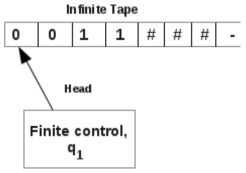A given transition is, δ(q1, 0) = (q2, x, R)
Then, TM becomes,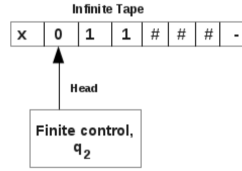A given transition is, δ(q2, 0) = (q2, 0, R)
Then, TM becomes,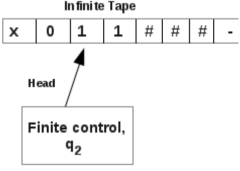A given transition is, δ(q2, 1) = (q3, y, L)
Then, TM becomes,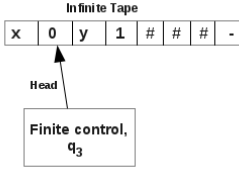A given transition is, δ(q3, 0) = (q4, 0, L)
Then, TM becomes,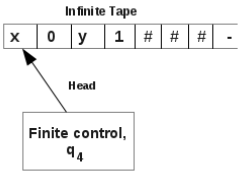A given transition is, δ(q4, x) = (q1, x, R)
Then, TM becomes,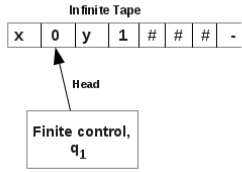A given transition is, δ(q1, 0) = (q2, x, R)
Then, TM becomes,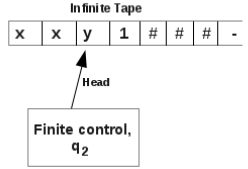A given transition is, δ(q2, y) = (q2, y, R)
Then, TM becomes,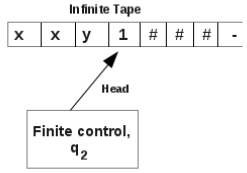A given transition is, δ(q2, 1) = (q3, y, L)
Then, TM becomes,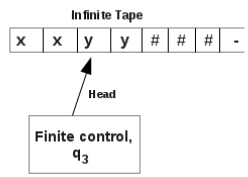A given transition is, δ(q3, y) = (q3, y, L)
Then, TM becomes,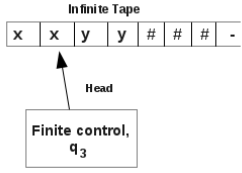A given transition is, δ(q3, x) = (q5, x, R)
Then, TM becomes,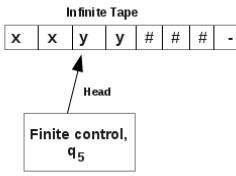A given transition is, δ(q5, y) = (q5, x, R)
Then, TM becomes,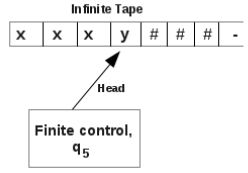A given transition is, δ(q5, y) = (q5, x, R)
Then, TM becomes,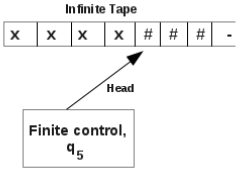A given transition is, δ(q5, #) = (q6, #, R)
Then, TM becomes,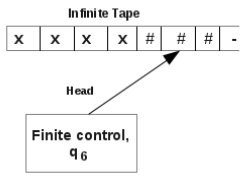qis the halt state or accepting state of the TM. So the string 0011 is accepted by the above TM.

Example 3:
Consider a Turing machine,
TM = (Q,∑, Γ, δ, q0, #, h)
where
Q = {q1, q2, q3, q4, q5, q6}
∑= {0, 1, x, y}
Γ = {0, 1, x, y, #}
q0 = q1
# = #
h = q6
δ is defined as,
δ(q1, 0) = (q2, x, R)     δ(q1, #) = (q5, #, R)
δ(q2, 0) = (q2, 0, R)     δ(q2, 1) = (q3, y, L)
δ(q2, y) = (q2, y, R)     δ(q3, 0) = (q4, 0, L)
δ(q3, x) = (q5, x, R)     δ(q3, y) = (q3, y, L)
δ(q4, 0) = (q4, 0, L)     δ(q4, x) = (q1, x, R)
δ(q5, y) = (q5, x, R)     δ(q5, #) = (q6, #, R)

Check whether the string 001 is accepted by the above TM?

Initially, TM is in start state, q1 and the tape contains the given string 001. Initially, head points to symbol 0 in the inputstring.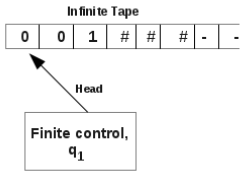A given transition is, δ(q1, 0) = (q2, x, R)
Then, TM becomes,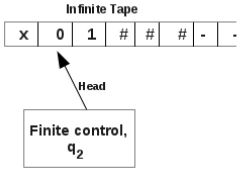A given transition is, δ(q2, 0) = (q2, 0, R)
Then, TM becomes,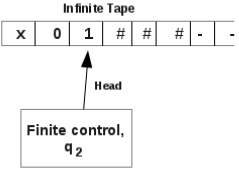A given transition is, δ(q2, 1) = (q3, y, L)
Then, TM becomes,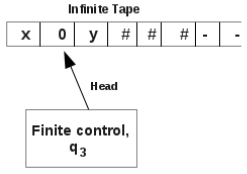A given transition is, δ(q3, 0) = (q4, 0, L)
Then, TM becomes,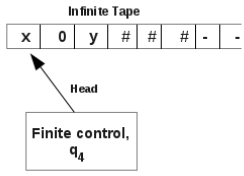A given transition is, δ(q4, x) = (q1, x, R)
Then, TM becomes,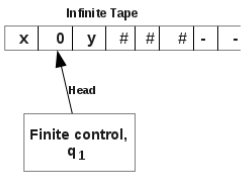A given transition is, δ(q1, 0) = (q2, x, R)
Then, TM becomes,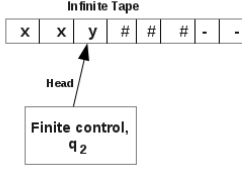A given transition is, δ(q2, y) = (q2, y, R)
Then, TM becomes,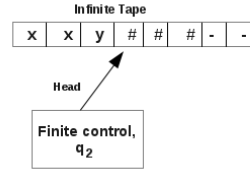Now TM halts because no δ is defined for q2 and #. But q2 is not the halt state of TM. So the string 001 is not accepted
by the above TM.

2 Representation of Turing Machines
We can represent a TM in different ways. They are,
Instantaneous descriptions,
Transition tables,
Transition diagrams.

Representation of TM by Instantaneous Descriptions
In all the above examples, Turing machines shown in the pictures were instaneous descriptions. This means an instant of a TM is shown.
Following shows an instantaneous description of a TM,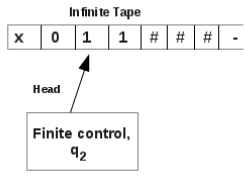Currently, symbol under the head is 1.
Current state is q2.
Symbols on the left of 1 are x and 0.
Symbnols on the right of 1 are 1, #, #, .....
This instant of TM can be written as,
x 0 q1 1 # # #.
Let a transition is defines as, δ(q2, 1) = (q3, y, L)
Then TM moves to,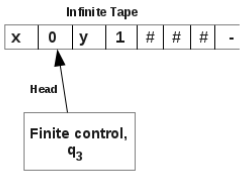That is,
x q2 0 y 1 # # #.
This transition from one state to other can be written as,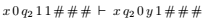Representation of TM by Transition Table
Let a Turing machine is defined as,
TM = (Q,∑, Γ, δ, q0, #, h)
where
Q = {q1, q2, q3, q4, q5, q6}
∑ = {0, 1, x, y}
Γ = {0, 1, x, y, #}
q0 = q1
# = #
h = q6

δ is defined as,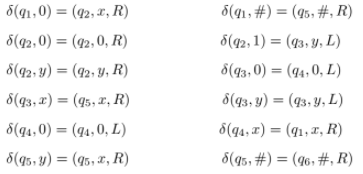This TM can be represented as a Transition table.
Transition table corresponding to the above TM is,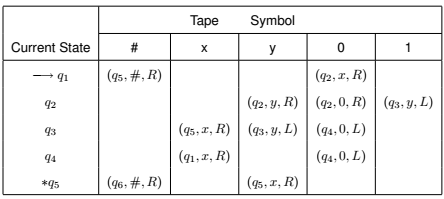Above is the transition table representation of a TM.
In the above transition table, the entry, (q2, y, R) corresponding to row q2 and column y denotes the transition,
δ(q2, y) = (q2, y, R)

Representation of TM by Transition Diagram
Let a Turing machine is defined as,
TM = (Q,∑, Γ, δ, q0, #, h)
where
Q = {q1, q2, q3, q4, q5, q6}
∑ = {0, 1, x, y}
Γ = {0, 1, x, y, #}
q0 = q1
# = #
h = q6
δ is defined as,
δ(q1, 0) = (q2, x, R)      δ(q1, #) = (q5, #, R)
δ(q2, 0) = (q2, 0, R)     δ(q2, 1) = (q3, y, L)
δ(q2, y) = (q2, y, R)      δ(q3, 0) = (q4, 0, L)
δ(q3, x) = (q5, x, R)     δ(q3, y) = (q3, y, L)
δ(q4, 0) = (q4, 0, L)      δ(q4, x) = (q1, x, R)
δ(q5, y) = (q5, x, R)      δ(q5, #) = (q6, #, R)

This TM can be represented as a Transition diagram.
Transition diagram corresponding to the above TM is,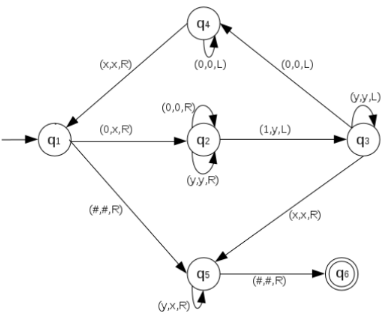In the above transition diagram, the part of th picture,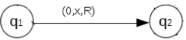denotes the transition,
δ(q1, 0) = (q2, x, R)
Also the part of the picture,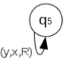denotes the transition,
δ(q5, y) = (q5, x, R)

Exercises:
1. Consider the TM given in the following transition table: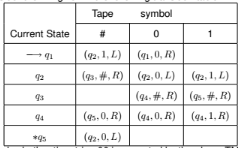Check whether the string 00 is accepted by the above TM.

2. Consider the TM given in the following transition table: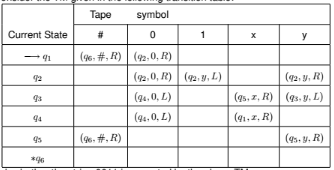Check whether the string 0011 is accepted by the above TM.

Offer running on EduRev: Apply code STAYHOME200 to get INR 200 off on our premium plan EduRev Infinity!

## Theory of Computation

18 videos|44 docs|39 tests

,

,

,

,

,

,

,

,

,

,

,

,

,

,

,

,

,

,

,

,

,

;